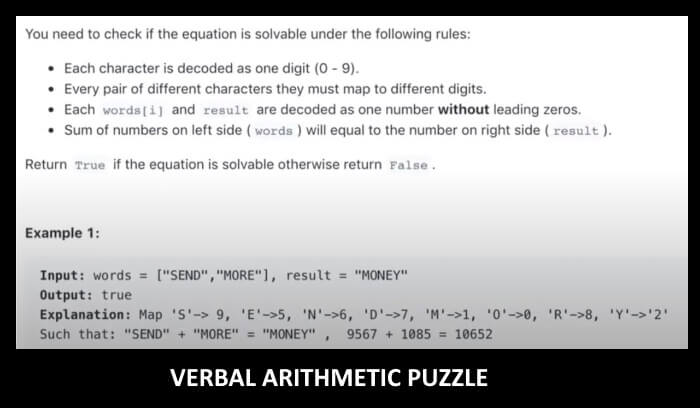Posted on

# Verbal Arithmetic PuzzlesLet’s do maths with letters.

What are Verbal Arithmetic Puzzles

This type of puzzles belongs to the recreational arithmetic domain and they’re basically equations in which you have to determine the value attributed to each letter. Obviously, all the number in such a puzzle will have to be a digit between 0 and 9.

Let’s have a look at one of the most famous puzzles of this type, which was published almost a century ago, in 1924, in the ‘Standard Magazine’

S E N D  +

M O R E  =

M O N E Y

It’s not puzzling at all if you know a little math. The first clue is the M, which as a carry-over can only be 1, which indicates next that O can only be 0, S must be nine and so on.

Here’s another easy puzzle for you:

S U N +

T A N=

B U R N

As you’ve seen in the first example, B can only be 1. Can you figure out the solution?

Once you have assigned a value to a character replace it wherever it appears in the puzzle and try to figure out the rest of the problem.

Most people will solve verbal arithmetic puzzles by hand, except for mathematicians who love to make everything more complicated and use terms like algorithms and integers.

See our in-house puzzle created by Animatopica

Watch Video

Types of Verbal Arithmetic Puzzles

The puzzles that use letters for numbers are called alphametics. These are sometimes combined with Sudoku or Kakuro puzzles, to create some fiendishly cryptic puzzles.

Puzzles in which a digit stands for another are known as digimetics.

Skeletal divisions are puzzles involving long divisions in which the numbers are replaced by symbols.

History of Verbal Arithmetic Puzzles

It’s impossible to say when arithmetic puzzles were invented, probably long ago. What we do know is that the term “cryptarithm” was coined in 1931. In 1942, this was changed to “cryptarithmetic”, which is a bit more clear.

See our in-house puzzle created by Animatopica